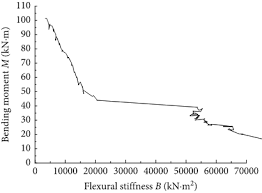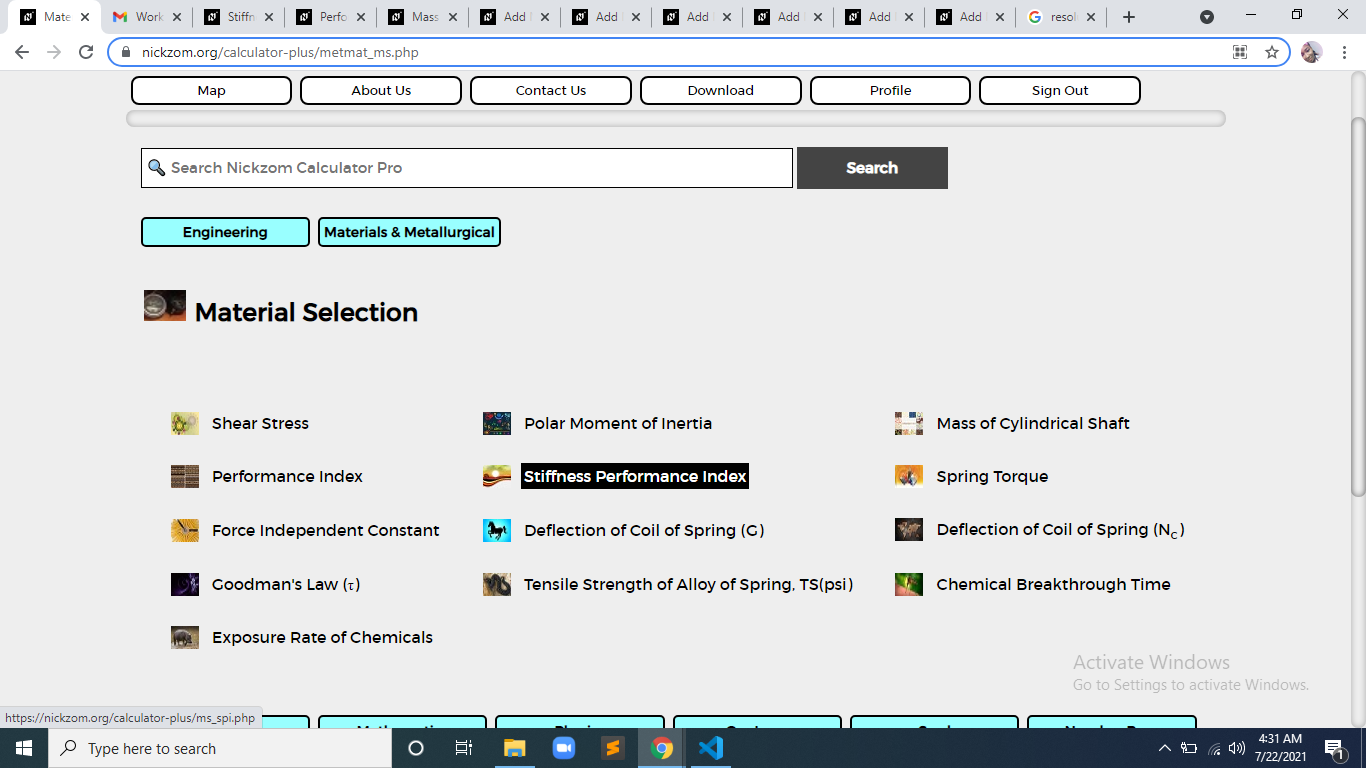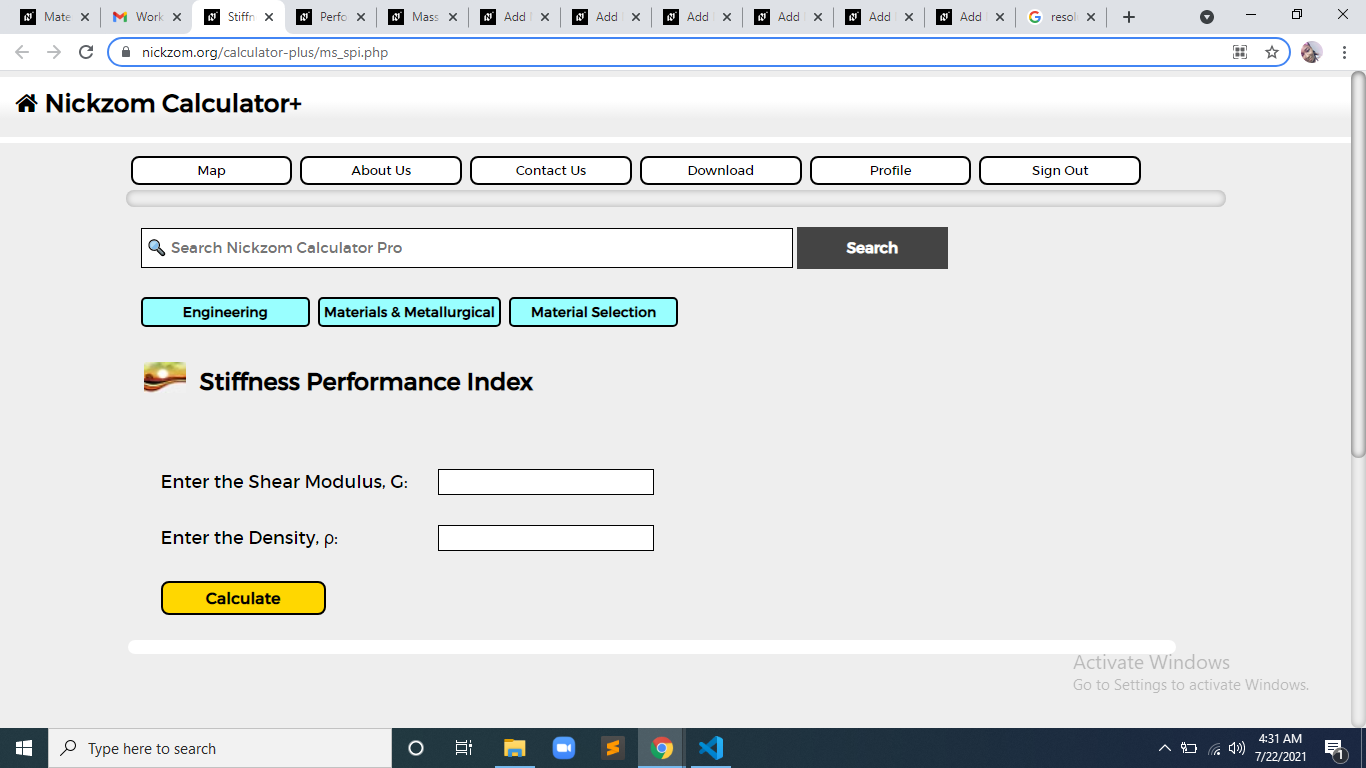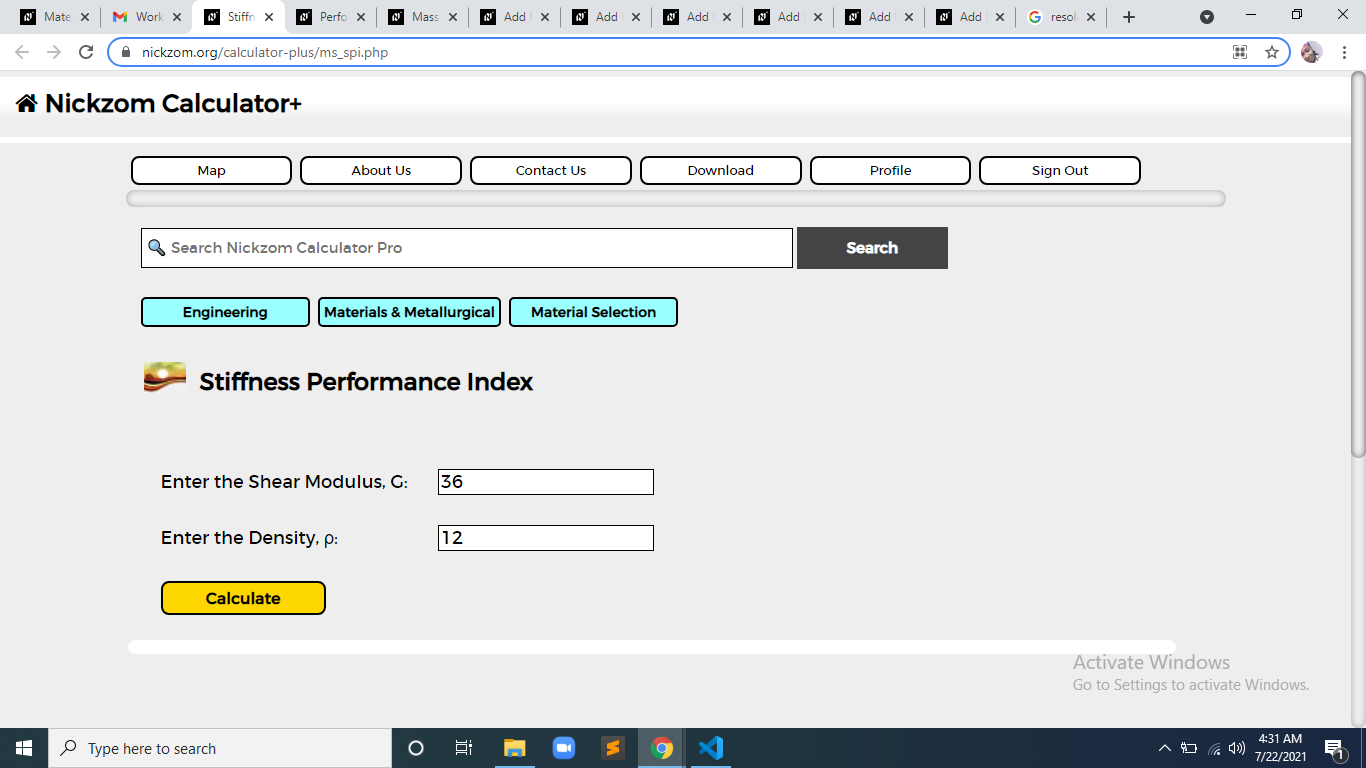# How to Calculate and Solve for Stiffness Performance Index | Material SelectionThe image above represents stiffness performance index.

To compute for stiffness performance index, two essential parameters are needed and these parameters are Shear Modulus (G) and Density (ρ).

The formula for calculating stiffness performance index:

P = √(G)/ρ

Where:

P = Stiffness Performance Index
G = Shear Modulus
ρ = Density

Let’s solve an example;
Find the stiffness performance index when the shear modulus is 36 and the density is 12.

This implies that;

G = Shear Modulus = 36
ρ = Density = 12

P = √(G)/ρ
P = √(36)/12
P = 6/12
P = 0.5

Therefore, the stiffness performance index is 0.5

Calculating the Shear Modulus when the Stiffness Performance Index and the Density is Given.

G = (P x ρ)2

Where:

G = Shear Modulus
P = Stiffness Performance Index
G = Shear Modulus= Stiffness Performance Index
ρ = Density

Let’s solve an example;
Find the shear modulus when the stiffness performance index is 25 and the density is 5.

This implies that;

P = Stiffness Performance Index = 25
ρ = Density = 5

G = (P x ρ)2
G = (25 x 5)2
G = 15,625

Therefore, the shear modulus is 15,625.

Calculating the Density when the Stiffness Performance Index and Shear Modulus is Given.

ρ = √(G) / P

Where;

ρ = Density
P = Stiffness Performance Index
G = Shear Modulus

Let’s solve an example;
Find the density when the stiffness performance index is 4 and the shear modulus is 64.

This implies that;

P = Stiffness Performance Index = 4
G = Shear Modulus = 64

ρ = √(G) / P
ρ = √64 / 4
ρ = 8 / 4
ρ = 2

Therefore, the density is 2.

Nickzom Calculator – The Calculator Encyclopedia is capable of calculating the stiffness performance index.

To get the answer and workings of the stiffness performance index using the Nickzom Calculator – The Calculator Encyclopedia. First, you need to obtain the app.

You can get this app via any of these means:

To get access to the professional version via web, you need to register and subscribe for NGN 2,000 per annum to have utter access to all functionalities.
You can also try the demo version via https://www.nickzom.org/calculator

Apple (Paid) – https://itunes.apple.com/us/app/nickzom-calculator/id1331162702?mt=8
Once, you have obtained the calculator encyclopedia app, proceed to the Calculator Map, then click on Materials and Metallurgical under Engineering.Now, Click on Material Selection under Materials and MetallurgicalNow, Click on Stiffness Performance Index under Material SelectionThe screenshot below displays the page or activity to enter your values, to get the answer for the stiffness performance index according to the respective parameter which is the Shear Modulus (G) and Density (ρ).Now, enter the values appropriately and accordingly for the parameters as required by the Shear Modulus (G) is 36 and Density (ρ) is 12.Finally, Click on CalculateAs you can see from the screenshot above, Nickzom Calculator– The Calculator Encyclopedia solves for the stiffness performance index and presents the formula, workings and steps too.